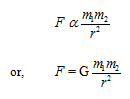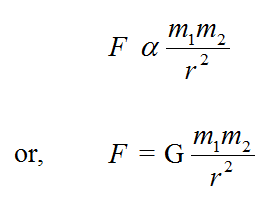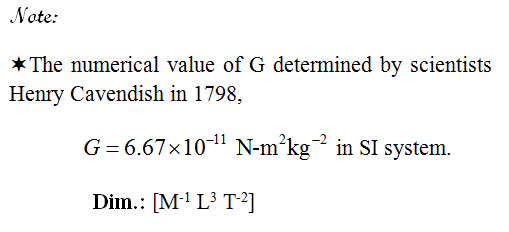# Gravitation

## Gravitation

#### Gravitation

Everybody in this universe attracts each other body with a force called force of gravitation. The force of attraction between a chair and a table lying in your classroom, the force of attraction between you and your friend etc. are example of gravitation. Gravity is a special case of gravitation. If one of the two attracting bodies is comparatively very large like moon, earth or any other planet then the force of attraction between the two bodies is called gravity. Gravitation is always attractive in nature.

#### Kepler’s Laws of planetary Motion:

1. Law of orbits (First law): All planets revolve in elliptical orbits around the sun and the sun is situated at one of its foci of the elliptical path.

2. Law of area (second law): The areal velocity of a planet remains constant, i.e., the line joining the sun to planet covers equal areas in same interval of time.

3. Law of periods (third law): The Square of the period of revolution of the planet is directly proportional to the cube of the semi major axis of its orbit.Newton’s Universal Law of Gravitation:
On the basis of Kepler’s laws of planetary motion, Newton stated his famous law of gravitation. According to this law:
Every two objects in the universe attract each other and the force of attraction between the bodies is directly proportional to the product of their masses and inversely proportional to the square of the distance between them.
i.e.,